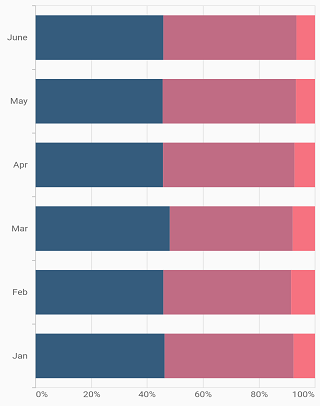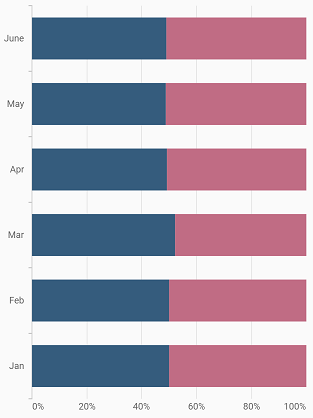# 100% Stacked bar Chart in Flutter Cartesian Charts (SfCartesianChart)

11 Apr 202210 minutes to read

To create a Flutter 100% stacked bar chart quickly, you can check this video.

To render a 100% stacked bar chart, create an instance of `StackedBar100Series`, and add it to the `series` collection property of `SfCartesianChart`. The following properties can be used to customize the appearance:

``````@override
Widget build(BuildContext context) {
return Scaffold(
body: Center(
child: Container(
child: SfCartesianChart(
primaryXAxis: CategoryAxis(),
series: <ChartSeries>[
StackedBar100Series<ChartData, String>(
dataSource: chartData,
xValueMapper: (ChartData data, _) => data.x,
yValueMapper: (ChartData data, _) => data.y
),
StackedBar100Series<ChartData, String>(
dataSource: chartData,
xValueMapper: (ChartData data, _) => data.x,
yValueMapper: (ChartData data, _) => data.y2
),
StackedBar100Series<ChartData, String>(
dataSource: chartData,
xValueMapper: (ChartData data, _) => data.x,
yValueMapper: (ChartData data, _) => data.y3
),
StackedBar100Series<ChartData, String>(
dataSource: chartData,
xValueMapper: (ChartData data, _) => data.x,
yValueMapper: (ChartData data, _) => data.y4
)
]
)
)
)
);
}``````## Bar width and spacing

The `spacing` property is used to change the spacing between two segments. The default value of spacing is 0, and the value ranges from 0 to 1. Here, 1 and 0 correspond to 100% and 0% of the available space, respectively.

The `width` property is used to change the width of the rectangle. The default value of the width is 0.7, and the value ranges from 0 to 1. Here, 1 and 0 correspond to 100% and 0% of the available width, respectively.

``````@override
Widget build(BuildContext context) {
return Scaffold(
body: Center(
child: Container(
child: SfCartesianChart(
primaryXAxis: CategoryAxis(),
series: <ChartSeries>[
StackedBar100Series<ChartData, String>(
dataSource: chartData,
xValueMapper: (ChartData data, _) => data.x,
yValueMapper: (ChartData data, _) => data.y,
width: 0.8,
spacing: 0.2
),
StackedBar100Series<ChartData, String>(
dataSource: chartData,
xValueMapper: (ChartData data, _) => data.x,
yValueMapper: (ChartData data, _) => data.y2,
width: 0.8,
spacing: 0.2
)
]
)
)
)
);
}``````